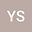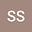Multiple solutions for a class of non-cooperative critical nonlocal equation system with variable exponents
••• Yueqiang Song,
• Shaoyun Shi
Yueqiang Song
Changchun Normal University
Author ProfileShaoyun Shi
Jilin University
Author Profile## Abstract

In this paper, we consider a class of non-cooperative critical nonlocal equation system with variable exponents of the form: $$\left\{ \begin{array}{lll} -(-\Delta)_{p(\cdot,\cdot)}^su - |u|^{p(x)-2}u = F_u(x,u,v) + |u|^{q(x)-2}u, \quad &\mbox{in}\,\,\mathbb{R}^N,\\ (-\Delta)_{p(\cdot,\cdot)}^sv + |v|^{p(x)-2}v = F_v(x,u,v) + |v|^{q(x)-2}u, \quad &\mbox{in}\,\,\mathbb{R}^N,\\ u, v \in W^{s,p(\cdot,\cdot)}(\mathbb{R}^N), \end{array}\right.$$ where $\nabla F = (F_u, F_v)$ is the gradient of a $C^1$-function $F: \mathbb{R}^N\times \mathbb{R}^2 \rightarrow \mathbb{R}^+$ with respect to the variable $(u, v) \in \mathbb{R}^2$. We also assume that$\{x \in \mathbb{R}^N: q(x) = p_s^\ast(x)\} \neq \emptyset$, here $p_s^\ast(x)=Np(x,x)/(N-sp(x,x))$ is the critical Sobolev exponent for variable exponents. With the help of the Limit index theory and the concentration-compactness principles for fractional Sobolev spaces with variable exponents, we establish the existence of infinitely many solutions for the problem under the suitable conditions on the nonlinearity.

#### Peer review status:UNDER REVIEW

06 Aug 2020Submitted to Mathematical Methods in the Applied Sciences
07 Aug 2020Assigned to Editor
07 Aug 2020Submission Checks Completed
16 Aug 2020Reviewer(s) Assigned